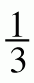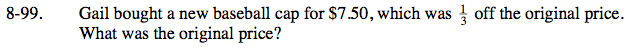### Home > CC2 > Chapter 8 > Lesson 8.3.4 > Problem8-99

8-99.

Gail bought a new baseball cap for $7.50, which wasoff the original price. What was the original price? Homework Help ✎$\text{If the new baseball cap is }\frac{1}{3}\text{ off the original price, then it must be }\frac{2}{3} \text{ of the original price.}$ Write an equation to represent the new cap's price as a fraction of the old cap's original price. $(7.50) = \frac{2}{3} x$ x =$11.25